# Dickman function

(diff) ← Older revision | Latest revision (diff) | Newer revision → (diff)

The function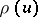defined on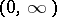by the initial condition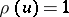forand by the delay-differential equation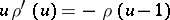for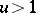. Interest attaches to this function because of its connection to "smooth" numbers, i.e. numbers that are the product of many small prime numbers. Letdenote the number of positive integers less than or equal toand free of prime divisors greater than. Whenis much larger than, it is a simple matter of inclusion-and-exclusion counting (cf. also Inclusion-exclusion formula) to show that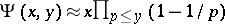, wheredenotes a prime number. But the error terms grow rapidly, and the "main" term gives the wrong answer in the ranges of greatest interest, including the case whenis comparable to a fixed fractional power of. For this case, K. Dickman found that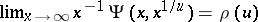. If, in place of the restriction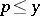for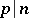one takes the condition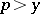, the resulting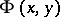is approximated by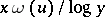, whereis the Bukhstab function, defined by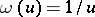,, and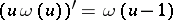,, where forthe right-hand derivative has to be taken, [a1]. Unlike,oscillates and tends to a positive limit, equal to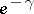.

There are two combinatorial identities linking the Dickman function to. Early work is based on the Bukhstab identity: Withdenoting a prime number, for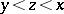,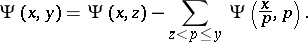The usual heuristic device of replacing a sum over prime numbers by an integral with "prime density"and replacingwith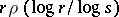leads to an identity which, when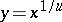and, simplifies to an integral equivalent to the definition of. N.G. de Bruijn carried this idea to its limits in [a2], but accuracy suffers when large and comparable estimated quantities must be subtracted. The more recent Hildebrand identity involves only additions and has the further advantage that the second input is the samethroughout: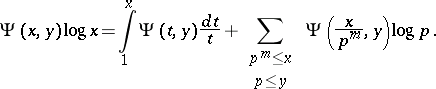Applications require estimates uniform in; the best known estimate along these lines, due to A. Hildebrand and based on the identity above, isuniformly in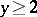and. There are similar results for algebraic integers, [a3].

There are also results concerning the number of smooth integers in an interval, and concerning the distribution of smooth integers into congruence classes [a4], [a5].

The Riemann hypothesis (cf. Riemann hypotheses) implies[a8].

The analytical properties ofare reasonably well understood; calculus, analysis of the Laplace transform, and the saddle-point method are the key tools. The first extensive analysis is due to De Bruijn, and the functionis sometimes termed the Dickman–De Bruijn function. One has [a10]:

a)for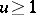(so thatis positive for all positive, and hence, from the definition, decreasing);

b)is log-concave, that is,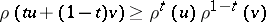for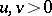and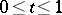(K. Alladi);

c) The Laplace transform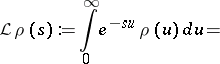d)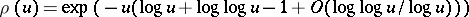as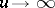.

The Dickman function is one of a parameterized family of related functions, [a12], and a wider class of similar delay-differential equations has been studied in [a7]. A quick and simple bit of Mathematica code suffices to calculateto reasonable accuracy in the intervalusing step-size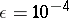. This code calculates a table of values ofat intervals of length, working back recursively into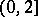:'

<tbody> </tbody>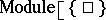,;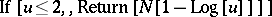;[a1] N.G. de Bruijn, "On the number of positive integers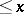and free prime factors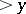. II" Indag. Math. , 28 (1966) pp. 239–247 Nederl. Akad. Wetensch. Proc. Ser. A , 69 (1966) [a2] N.G. de Bruijn, "On the number of uncancelled elements in the sieve of Eratosthenes" Indag. Math. , 12 (1950) pp. 247–256 Nederl. Akad. Wetensch. Proc. , 53 (1950) pp. 803–812 [a3] J. Friedlander, "On the number of ideals free from large prime divisors" J. Reine Angew. Math. , 255 (1972) pp. 1–7 [a4] J. Friedlander, A. Granville, "Integers without large prime factors, in short intervals" Philos. Trans. Royal Soc. , 345 (1993) pp. 339–348 [a5] A. Granville, "On integers, without large prime factors, in arithmetic progressions II" Philos. Trans. Royal Soc. , 345 (1993) pp. 349–362 [a6] A. Hildebrand, G. Tenenbaum, "Integers without large prime factors" J. Théor. Nombres Bordeaux , 5 : 2 (1993) pp. 411–484 [a7] A. Hildebrand, G. Tenenbaum, "On a class of differential-difference equations arising in number theory" J. Anal. Math. , 61 (1993) pp. 145–179 [a8] A. Hildebrand, "Integers free of large prime factors and the Riemann hypothesis" Mathematika , 31 : 2 (1985) pp. 258–271 [a9] S. Hunter, J. Sorenson, "Approximating the number of integers free of large prime factors" Math. Comput. , 66 : 220 (1997) pp. 1729–1741 [a10] P. Moree, "Psixyology and Diophantine equations" Diss. Univ. Leiden (1993) [a11] E. Saias, "Sur le nombre des entiers sans grand facteur premier" J. Number Theory , 32 : 1 (1989) pp. 78–99 [a12] F.S. Wheeler, "Two differential-difference equations arising in number theory" Trans. Amer. Math. Soc. , 318 : 2 (1990) pp. 491–523 [a13] D.S. Mitrinović, J. Sandor, B. Crstici, "Handbook of number theory" , Kluwer Acad. Publ. (1996) pp. Sect. IV.21 [a14] K. Dickman, "On the frequency of numbers containing prime factors of a certain relative magnitude" Ark. Mat., Astron. och Fysik , 22A : 10 (1930) pp. 1–14
How to Cite This Entry:
Dickman function. Encyclopedia of Mathematics. URL: http://encyclopediaofmath.org/index.php?title=Dickman_function&oldid=17561
This article was adapted from an original article by D. Hensley (originator), which appeared in Encyclopedia of Mathematics - ISBN 1402006098. See original article